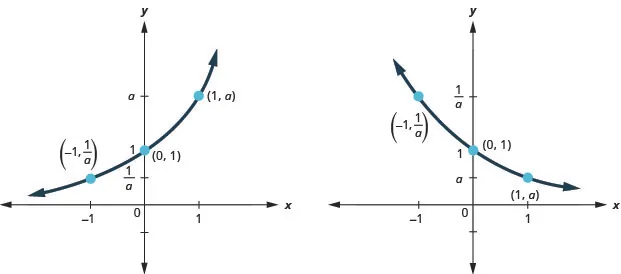Intermediate Algebra

# Key Concepts

Intermediate AlgebraKey Concepts

### Key Concepts

#### 10.1Finding Composite and Inverse Functions

• Composition of Functions: The composition of functions $ff$ and $g,g,$ is written $f∘gf∘g$ and is defined by
$(f∘g)(x)=f(g(x))(f∘g)(x)=f(g(x))$

We read $f(g(x))f(g(x))$ as $ff$ of $gg$ of $x.x.$
• Horizontal Line Test: If every horizontal line, intersects the graph of a function in at most one point, it is a one-to-one function.
• Inverse of a Function Defined by Ordered Pairs: If $f(x)f(x)$ is a one-to-one function whose ordered pairs are of the form $(x,y),(x,y),$ then its inverse function $f−1(x)f−1(x)$ is the set of ordered pairs $(y,x).(y,x).$
• Inverse Functions: For every $xx$ in the domain of one-to-one function $ff$ and $f−1,f−1,$
$f−1(f(x))=xf(f−1(x))=xf−1(f(x))=xf(f−1(x))=x$
• How to Find the Inverse of a One-to-One Function:
1. Step 1. Substitute y for $f(x).f(x).$
2. Step 2. Interchange the variables x and y.
3. Step 3. Solve for y.
4. Step 4. Substitute $f−1(x)f−1(x)$ for $y.y.$
5. Step 5. Verify that the functions are inverses.

#### 10.2Evaluate and Graph Exponential Functions

• Properties of the Graph of $f(x)=ax:f(x)=ax:$
when $a>1a>1$ when $0
Domain $(−∞,∞)(−∞,∞)$ Domain $(−∞,∞)(−∞,∞)$
Range $(0,∞)(0,∞)$ Range $(0,∞)(0,∞)$
$xx$-intercept none $xx$-intercept none
$yy$-intercept $(0,1)(0,1)$ $yy$-intercept $(0,1)(0,1)$
Contains $(1,a),(−1,1a)(1,a),(−1,1a)$ Contains $(1,a),(−1,1a)(1,a),(−1,1a)$
Asymptote $xx$-axis, the line $y=0y=0$ Asymptote $xx$-axis, the line $y=0y=0$
Basic shape increasing Basic shape decreasing• One-to-One Property of Exponential Equations:
For $a>0a>0$ and $a≠1,a≠1,$
$A=A0ertA=A0ert$
• How to Solve an Exponential Equation
1. Step 1. Write both sides of the equation with the same base, if possible.
2. Step 2. Write a new equation by setting the exponents equal.
3. Step 3. Solve the equation.
4. Step 4. Check the solution.
• Compound Interest: For a principal, $P,P,$ invested at an interest rate, $r,r,$ for $tt$ years, the new balance, $A,A,$ is
$A=P(1+rn)ntwhen compoundedntimes a year. A=Pertwhen compounded continuously. A=P(1+rn)ntwhen compoundedntimes a year. A=Pertwhen compounded continuously.$
• Exponential Growth and Decay: For an original amount, $A0A0$ that grows or decays at a rate, $r,r,$ for a certain time $t,t,$ the final amount,$A,A,$ is $A=A0ert.A=A0ert.$

#### 10.3Evaluate and Graph Logarithmic Functions

• Properties of the Graph of $y=logax:y=logax:$
when $a>1a>1$ when $0
Domain $(0,∞)(0,∞)$ Domain $(0,∞)(0,∞)$
Range $(−∞,∞)(−∞,∞)$ Range $(−∞,∞)(−∞,∞)$
x-intercept $(1,0)(1,0)$ x-intercept $(1,0)(1,0)$
y-intercept none y-intercept none
Contains $(a,1),(a,1),$$(1a,−1)(1a,−1)$ Contains $(a,1),(a,1),$$(1a,−1)(1a,−1)$
Asymptote y-axis Asymptote y-axis
Basic shape increasing Basic shape decreasing• Decibel Level of Sound: The loudness level, $DD$, measured in decibels, of a sound of intensity, $II$, measured in watts per square inch is $D=10log(I10−12).D=10log(I10−12).$
• Earthquake Intensity: The magnitude $RR$ of an earthquake is measured by $R=logI,R=logI,$ where $II$ is the intensity of its shock wave.

#### 10.4Use the Properties of Logarithms

• Properties of Logarithms
$loga1=0logaa=1loga1=0logaa=1$
• Inverse Properties of Logarithms
• For $a>0,a>0,$$x>0x>0$ and $a≠1a≠1$
$alogax=xlogaax=xalogax=xlogaax=x$
• Product Property of Logarithms
• If $M>0,N>0,a>0M>0,N>0,a>0$ and $a≠1,a≠1,$ then,
$logaM·N=logaM+logaNlogaM·N=logaM+logaN$

The logarithm of a product is the sum of the logarithms.
• Quotient Property of Logarithms
• If $M>0,N>0,a>0M>0,N>0,a>0$ and $a≠1,a≠1,$ then,
$logaMN=logaM−logaNlogaMN=logaM−logaN$

The logarithm of a quotient is the difference of the logarithms.
• Power Property of Logarithms
• If $M>0,a>0,a≠1M>0,a>0,a≠1$ and $pp$ is any real number then,
$logaMp=plogaMlogaMp=plogaM$

The log of a number raised to a power is the product of the power times the log of the number.
• Properties of Logarithms Summary
If $M>0,a>0,a≠1M>0,a>0,a≠1$ and $pp$ is any real number then,
Property Base $aa$ Base $ee$
$loga1=0loga1=0$ $ln1=0ln1=0$
$logaa=1logaa=1$ $lne=1lne=1$
Inverse Properties $alogax=x logaax=xalogax=x logaax=x$ $elnx=x lnex=xelnx=x lnex=x$
Product Property of Logarithms $loga(M·N)=logaM+logaNloga(M·N)=logaM+logaN$ $ln(M·N)=lnM+lnNln(M·N)=lnM+lnN$
Quotient Property of Logarithms $logaMN=logaM−logaNlogaMN=logaM−logaN$ $lnMN=lnM−lnNlnMN=lnM−lnN$
Power Property of Logarithms $logaMp=plogaMlogaMp=plogaM$ $lnMp=plnMlnMp=plnM$
• Change-of-Base Formula
For any logarithmic bases a and b, and $M>0,M>0,$
$logaM=logbMlogbalogaM=logMlogalogaM=lnMlna new basebnew base 10new baseelogaM=logbMlogbalogaM=logMlogalogaM=lnMlna new basebnew base 10new basee$

#### 10.5Solve Exponential and Logarithmic Equations

• One-to-One Property of Logarithmic Equations: For $M>0,N>0,a>0,M>0,N>0,a>0,$ and $a≠1a≠1$ is any real number:
$IflogaM=logaN,thenM=N.IflogaM=logaN,thenM=N.$
• Compound Interest:
For a principal, P, invested at an interest rate, r, for t years, the new balance, A, is:
$A=P(1+rn)ntwhen compoundedntimes a year. A=Pertwhen compounded continuously.A=P(1+rn)ntwhen compoundedntimes a year. A=Pertwhen compounded continuously.$
• Exponential Growth and Decay: For an original amount, $A0A0$ that grows or decays at a rate, r, for a certain time t, the final amount, A, is $A=A0ert.A=A0ert.$
Order a print copy

As an Amazon Associate we earn from qualifying purchases.